Next: Magnetic susceptibility and permeability Up: Dielectric and magnetic media Previous: Energy density within a

## Magnetization

All matter is built up out of atoms, and each atom consists of electrons in motion. The currents associated with this motion are termed atomic currents. Each atomic current is a tiny closed circuit of atomic dimensions, and may therefore be appropriately described as a magnetic dipole. If the atomic currents of a given atom all flow in the same plane then the atomic dipole moment is directed normal to the plane (in the sense given by the right-hand rule), and its magnitude is the product of the total circulating current and the area of the current loop. More generally, if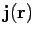is the atomic current density at the point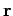then the magnetic moment of the atom is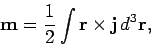(856)

where the integral is over the volume of the atom. If there aresuch atoms or molecules per unit volume then the magnetization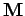(i.e., the magnetic dipole moment per unit volume) is given by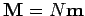. More generally,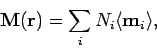(857)

where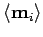is the average magnetic dipole moment of the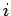th type of molecule in the vicinity of point, and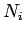is the average number of such molecules per unit volume at.

Consider a general medium which is made up of molecules which are polarizable and possess a net magnetic moment. It is easily demonstrated that any circulation in the magnetization field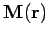gives rise to an effective current density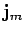in the medium. In fact,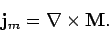(858)

This current density is called the magnetization current density, and is usually distinguished from the true current density,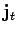, which represents the convection of free charges in the medium. In fact, there is a third type of current called a polarization current, which is due to the apparent convection of bound charges. It is easily demonstrated that the polarization current density,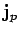, is given by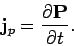(859)

Thus, the total current density,, in the medium takes the form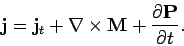(860)

It must be emphasized that all terms on the right-hand side of the above equation represent real physical currents, although only the first term is due to the motion of real charges (over more than atomic dimensions).

The differential form of Ampère's law is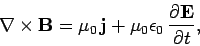(861)

which can also be written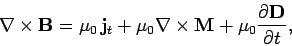(862)

where use has been made of the definition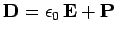. The above expression can be rearranged to give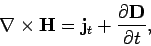(863)

where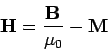(864)

is termed the magnetic intensity, and has the same dimensions as(i.e., magnetic dipole moment per unit volume). In a steady-state situation, Stokes' theorem tell us that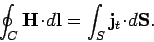(865)

In other words, the line integral of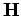around some closed curve is equal to the flux of true current through any surface attached to that curve. Unlike the magnetic field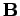(which specifies the force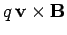acting on a charge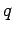moving with velocity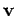), or the magnetization(the magnetic dipole moment per unit volume), the magnetic intensityhas no clear physical meaning. The only reason for introducing it is that it enables us to calculate fields in the presence of magnetic materials without first having to know the distribution of magnetization currents. However, this is only possible if we possess a constitutive relation connectingand.Next: Magnetic susceptibility and permeability Up: Dielectric and magnetic media Previous: Energy density within a
Richard Fitzpatrick 2006-02-02